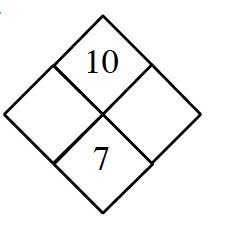### Home > AC > Chapter 3 > Lesson 3.1.7 > Problem3-68

3-68.

Copy and complete each of the Diamond Problems below. The pattern used in the Diamond Problems is shown at right.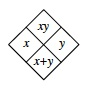1.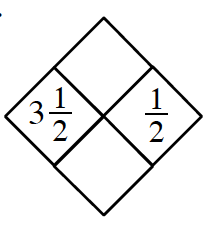Use the $x$ and $y$ values given to find the $xy$ value and $x+y$ value.

$\text{Top diamond: } 3\frac{1}{2} \cdot \frac{1}{2}$

$\text{Bottom diamond: } 3\frac{1}{2} + \frac{1}{2}$

1.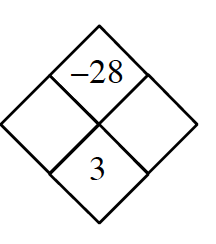Use the $x$ and $y$ values given to find the $xy$ value and $x+y$ value.

What numbers when multiplied equal $−28$ and when added equal $3$?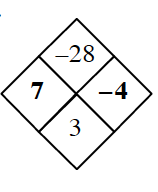1.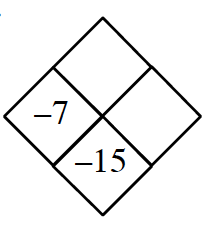See the hints in parts (a) and (b).

1.